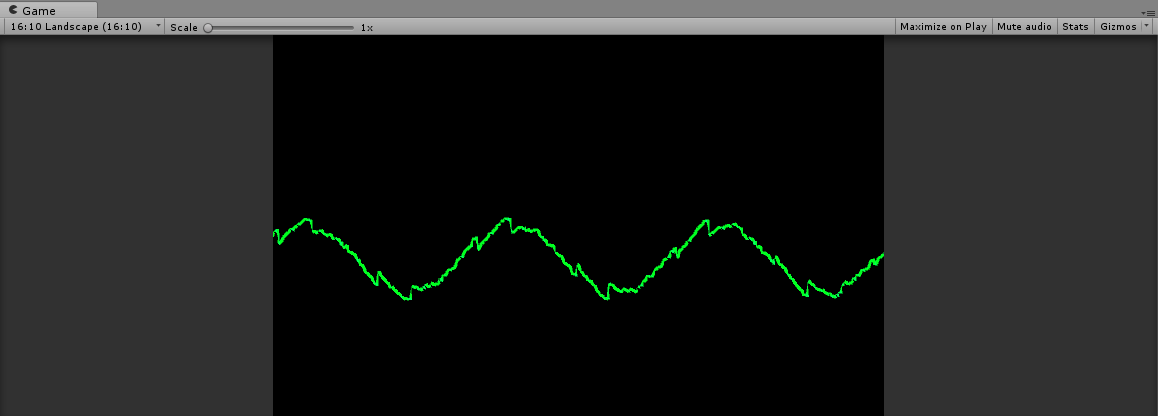## Audio Sound WaveNow that we have the simple data affecting the line renderer it’s time to make it resemble something closer to what a sound wave should look like. In order to achieve this it’s necessary to recognize what the data Unity is providing us from the AudioListener.

If we examine the raw data from a sample it appears to be very small values between 0 and 1 which tells us that this is the angular frequency of the sound at that specific sample. In basic Audio Wave Effect their were only lines being pushed up based on this data, what is actually being represented is a series of sine waves being viewed from their narrowest vantage meaning stacked together.

In order to decouple these sine waves we need to utilize the audio data in a new equation to form each individual wave. As such we begin by allocating an array of Vector3 for each point on the sine wave. Next we step through the audio data for the frame for each sine wave we wish to create, or rather for the number of samples our AudioSampler recordered. For each sample we loop over our array of Vector3 representing our final sound wave adding in the current samples sound wave using this formula:

fWave[j] += Vector3.up * gain * sample * Mathf.Sin((gain * sample * i * length * 2 * Mathf.PI) + dist);

Breaking apart this formula we have several operations going on, first we are obtaining the world up vector in order to make sure our result is a vector. Next we have the Amplitude represented as the gain multiplied by the sample. Finally we have the actual sine wave function with the angle being calculated as the gain  times the sample times the frequency times the length times 2 PI, then we do what is known as a phase shift using the distance this sample is from the start of our line.

Despite the bulky math this is a fairly physically accurate representation of an audio wave using the Unity Line Renderer. The final task is similar to previous effect in which we just pass the vertex data to our line renderer for that frame to be drawn to the screen.

```public class SynthesizerEffect : MonoBehaviour {
LineRenderer lRender;
AudioSampler aSampler;

[SerializeField]
Vector3 lStart, lEnd;
Vector3[] vArray;

[SerializeField]
[Range(1f, 10000f)]
float gain = 1f;
[SerializeField]
[Range(1, 64)]
int numWaves = 1;

int length = 0;

void Awake()
{
lRender = GetComponent<LineRenderer>();
}

void Start()
{
aSampler = AudioSampler.Instance;
if (aSampler == null || lRender == null)
{
Destroy(gameObject);
return;
}

length = aSampler.ArrayLength;
LineSetup();
}

internal void LineSetup()
{
float distance = (lStart - lEnd).magnitude / length;

Vector3 lVertex = lStart;
Vector3 offset = (lStart - lEnd).normalized * distance;

Vector3[] lVerts = new Vector3[length];
lRender.SetVertexCount(length);

for (int i = 0; i < length - 1; i++)
{
lVerts[i] = lVertex;
lVertex -= offset;
}

lVerts[length - 1] = lEnd;
lRender.SetPositions(lVerts);
vArray = lVerts;

}

void Update()
{
Vector3[] fWave = new Vector3[length];

for (int i = 0; i < numWaves; ++i)
{

float freq = aSampler.GetAudioData(i);
for (int j = 0; j < length; ++j)
{
var dist = (vArray - vArray[j]).magnitude;
fWave[j] += Vector3.up * gain * freq *
Mathf.Sin(
(gain * freq * i * length * 2 * Mathf.PI) + dist
);
}
}

for(int i=0; i < length; ++i)
{
lRender.SetPosition(
i,
vArray[i] + fWave[i] / length
);
}

}

}

```